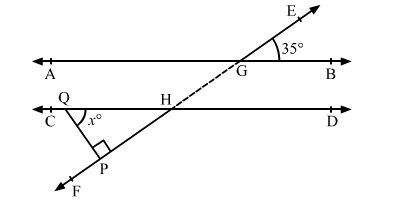# In the given figure, AB || CD and EF is a transversal, cutting them at G and H respectively.

Question:

In the given figure, AB || CD and EF is a transversal, cutting them at G and H respectively. If ∠EGB = 35° and QP ⊥ EF, find the measure of ∠PQH.Solution:

In the given figure, AB || CD and EF is a transversal.

∴ ∠PHQ = ∠EGB          (Pair of alternate exterior angles)

⇒ ∠PHQ = 35°

In ∆PHQ,

∠PHQ + ∠QPH + ∠PQH = 180°     (Angle sum property)

⇒ 35° + 90° + x = 180°

⇒ 125° + x = 180°

⇒ x = 180° − 125° = 55°

Thus, the measure of ∠PQH is 55°.